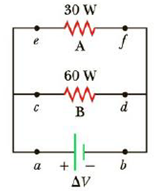Chapter 17.6, Problem 17.8QQ

Chapter
Section
Textbook Problem

For the two resistors shown in Figure 17.12, rank the currents at points a through f from largest to smallest. (a) Ia = Ib > Ie = If > Ic = Id (b) Ia= Ib > Ic = Id  > Ie = If  (c) Ie = If > Ic = Id > Ia = IbFigure 17.12 (Quick Quiz 17.8)

To determine
The order of currents at the marked points from largest to smallest.

Explanation

Given Info: Figure17.12

Explanation:

Conclusion: The current Ia splits into Ic and Ie . So, Ia>Ic>Ie . Since the resistor at A and B are connected in parallel with the voltage ΔV , voltage across the resistors are same. From the relation P=IΔV where P is the power delivered, I is the current and ΔV is the voltage across the resistor, the resistor with higher power rating carries more current. So Ic>Ie . By the law of conservation of charge, amount of charge flowed into a resistor must be equal to charge leave from the resistor, which means Ic=Id and Ie=If . The current leaving from the resistor recombines and goes into the battery

Still sussing out bartleby?

Check out a sample textbook solution.

See a sample solution

The Solution to Your Study Problems

Bartleby provides explanations to thousands of textbook problems written by our experts, many with advanced degrees!

Get Started